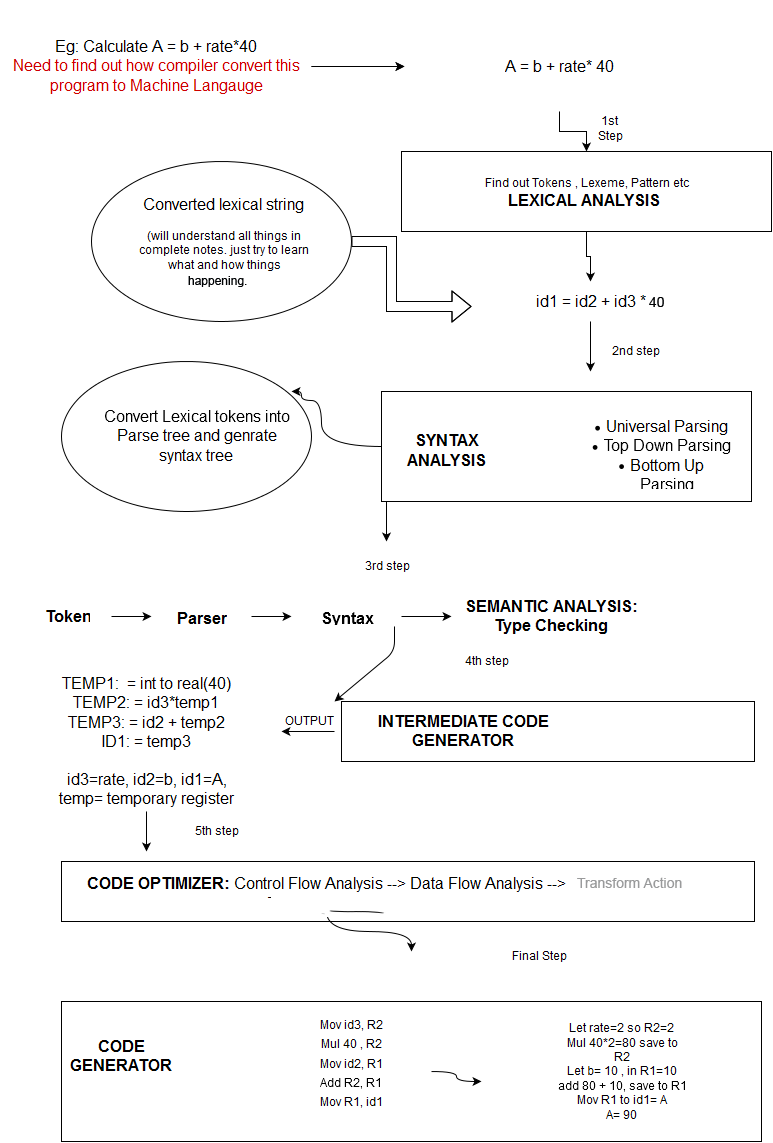## compiler design

Learn Single and Multi Pass Compiler , Learn Phases , Learn How compiler convert one program to Machine Lanaguage.
23 November, 2015
As we know Compiler has 6 parts and Each and every part has its own fundamental work.
To learn every thing follow the notes and carry one standard book and for practical we will use SPIM Simulator.
Before move to Phase one that is Lexical Analysis , we need to learn What is Pass:
1. All processing steps of compiler are grouped into two phases : Analysis and Synthesis.
2.    Analysis Part: Lexical, Syntax, Semantic, Intermediate Code Generator.
3.    Synthesis Part: Code Optimizer and Code Generator.
4.    Pass is the Processing performed by the Language Processor.
5.    First is Single Pass: Compile the program in single Pass.
6.    Multi Pass: Compile the program in multiple passes.
7.    Multi Pass compiler are also used when amount of memeory is limited.

Lets check out Front end and Back end Compiler phases:•  Front end consist that part of source language which is independent of target language.
•  Back end includes those portion of the compiler that depends on the target machine.
• Remember : C is a dependent language and JAVA is a independent language because the source file of C language is obtained by the help of Synthesis Phase while JAVA source file is obtained by Analysis Phase. Those languages which is obtained by analysis phase they don't need any specific platform to run , they can run any where with the help of source file.
•   Lexical Analysis: Main task to read program , produces sequence of tokens that the parser uses for syntax analysis.
•   Syntax Analysis: Assist programmer in identifying and locating errors by constructing syntax tree.
•   Semantic Analysis: Type checking , flow of control checks, uniqueness, name related check.
•   Intermediate Code Generator: Generating intermediate code for optimization.
•  Code Optimizer: Optimizing the intermediate code with the help of Three address codes and Parse tree.
•  Code Generator: Accurate, faster and target machine code builder.
•   Symbol table: Symbol table is a Data structure containing a record for each identifier with fields for he attributes of the identifier.
•   Error Handler: Handles all kinds of errors in each phase.
See what is exactly we are going to learn(see image):
•  Taking one example as a small program and will get machine code(binary form 0,1).
•  Calculate a = b + rate*40. Need to find machine code with the help of all Compiler phases.
•  In the very first step we get tokens, sequence of character with the help of Lexical Analysis(Complete part will you understand in rest of the coming lexical notes till then just understand we are breaking the programs into tokens.)
•  Regular expressions are widely used to specify patterns.
•  Output is id1 = id2 + id3* 40.
•  Syntax Analyzer : Generate parse tree.
•  Semantic Analyzer : Checking all errors and generate syntax tree for Intermediate code generate step.
•  Finally we get Intermediate codes: Temp1: = int to real(40)
Temp2 := id3*temp1(BODMAS rule )
Temp3 := id2+temp2
&nb•          Code generator: Generates Machine Code

Mov id3, R2

Mul 40, R2

Mov id2, R1

Mov R1, id1

Let rate=2 so R2=2                 // we have two temporary registers R1, R2[both active status=1]

Multiply 40*R2= 40*2=80     // multiply 40*2 and store in R2

Let b= 10 so R1=10                 // b=2 so register R1=10

Add 80 +10= 90 store in R1    // Add 80+10 and store in R1

Mov A=90&